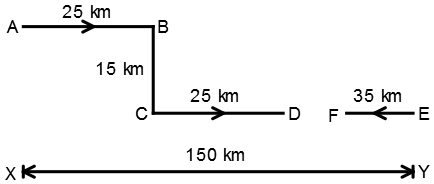# Two cars start from the opposite places of a main road, 150 km apart. First car runs for 25 km and takes a right turn and then runs 15 km. It then turns left and then runs for another 25 km and then takes the direction back to reach the main road. In the mean time, due to minor break down the other car has run only 35 km along the main road. What would be the distance between two cars at this point?

## Two cars start from the opposite places of a main road, 150 km apart. First car runs for 25 km and takes a right turn and then runs 15 km. It then turns left and then runs for another 25 km and then takes the direction back to reach the main road. In the mean time, due to minor break down the other car has run only 35 km along the main road. What would be the distance between two cars at this point?

A. 65 km
B. 75 km
C. 80 km
D. 85 km

Solution:Required Distance
= DF
= 150 – (25 + 25 + 35)
= 150 – 85
= 65 km